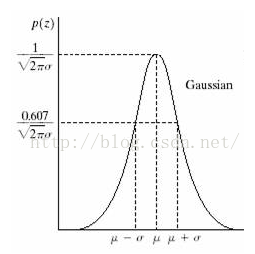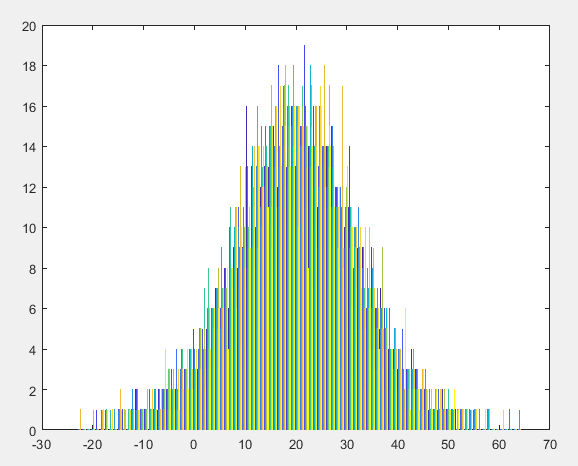# 图像处理均匀分布噪声

## 均匀分布噪声图像

2015-12-15 19:25:54 qq_18343569 阅读数 5417
• ###### 卷积神经网络实践技巧：数据增强、数据预处理、批归一化

128人学习 白勇
免费试看

(1) 如果,则称X服从离散的均匀分布。
(2) 设连续型随机变量X的概率密度函数为
f(x)=1/(b-a)，a≤x≤b

P{x1≤x≤x2}=(x2-x1)/(b-a)

### 分布函数

F(x)=0，x<a
F〔x〕= （x-a）/(b-a)，a≦x≦b。
F(x)=1，x>b

```clc,clear,close all
warning off
feature jit off
Z1 = imnoise_uniform(size(im,1),size(im,2),50,100);
Z1 = uint8(Z1);   % 类型转换
figure('color',[1,1,1]),
im(:,:,1) = im(:,:,1) + Z1;  % R
im(:,:,2) = im(:,:,2) + Z1;  % G
im(:,:,3) = im(:,:,3) + Z1;  % B
subplot(121); imshow(im); title('加均匀分布噪声图像')
subplot(122); imhist(Z1); title('均匀分布噪声图像直方图')

```

```function R = imnoise_uniform(M, N, a, b)
% input:
%       uniform噪声的类型；
%       M,N：输出噪声图像矩阵的大小
%       a,b：各种噪声的分布参数
% output:
%       R: 输出的噪声图像矩阵，数据类型为double型
% 设定默认值
if nargin == 1
a = 0; b = 1;
M = 1; N = 1;
elseif nargin == 3
a = 0; b = 1;
end

% 产生均匀分布噪声
R = a + (b - a)*rand(M, N);
end
```

## [数字图像处理]常见噪声的分类与Matlab实现

2014-07-11 16:45:12 thnh169 阅读数 40897

128人学习 白勇
免费试看

## 1.研究噪声特性的必要性

本文的内容主要介绍了常见噪声的分类与其特性。将噪声建模，然后用模型去实现各式各样的噪声。

实际生活中的各种照片的老化，都可以归结为以下老化模型。这个模型很简单，也可以直接用以下公式来表达。根据以上式子，可以看出，老旧照片的复原，主要分为两个任务，一个是去噪；另一个是去卷积，或者称为逆滤波，也就是将老化滤波器做反处理。

本文首先由噪声类型与其建模。随后的博文，会介绍几种基础的去噪方法和基础的逆滤波方法。

## 2.噪声的实现

### 2.1    评价用图像与其直方图### 2.2  高斯噪声

高斯噪声，也称为正态噪声，其统计特性服从正态分布。一种较为泛用的噪声模型。
Matlab的实现较为简单，Matlab已经有一个randn(M,N)的函数，用其可以产生出均值为0、方差为1、尺寸为M X N像素的高斯噪声图像。
用以下程序就可以产生任意均值和方差的高斯噪声。

```a = 0;
b = 0.08;
n_gaussian = a + b .* randn(M,N);```### 2.3 瑞利噪声

瑞利噪声相比高斯噪声而言，其形状向右歪斜，这对于拟合某些歪斜直方图噪声很有用。

瑞利噪声的实现可以借由平均噪声来实现。如下所示。```a = -0.2;
b = 0.03;
n_rayleigh = a + (-b .* log(1 - rand(M,N))).^0.5;```### 2.4 伽马噪声

伽马噪声的分布，服从了伽马曲线的分布。伽马噪声的实现，需要使用b个服从指数分布的噪声叠加而来。指数分布的噪声，可以使用均匀分布来实现。```a = 25;
b = 3;
n_Erlang = zeros(M,N);

for j=1:b
n_Erlang = n_Erlang + (-1/a)*log(1 - rand(M,N));
end```### 2.5 均匀噪声

如同前面所示，均匀噪声可以由函数rand(M,N)直接产生。```a = 0;
b = 0.3;
n_Uniform = a + (b-a)*rand(M,N);```### 2.6 椒盐噪声

椒盐噪声也成为双脉冲噪声。在早期的印刷电影胶片上，由于胶片化学性质的不稳定和播放时候的损伤，会使得胶片表面的感光材料和胶片的基底欠落，在播放时候，产生一些或白或黑的损伤。事实上，这也可以归结为特殊的椒盐噪声。

椒盐噪声的实现，需要一些逻辑判断。这里我们的思路是，产生均匀噪声，然后将超过阈值的点设置为黑点，或白点。当然，如果需要拟合电影胶片的损伤的话，可以选用别的类型噪声去拟合。

```a = 0.05;
b = 0.05;
x = rand(M,N);

g_sp = zeros(M,N);
g_sp = f;

g_sp(find(x<=a)) = 0;
g_sp(find(x > a & x<(a+b))) = 1;```## 3.总结

本文，实现的几类较为基本的噪声。并给出了其实现的方法，代码在下面。下一篇博文，会进行几个常用去噪滤波器的比较。

```close all;
clear all;
clc;

f = mat2gray(f,[0 255]);
[M,N] = size(f);

figure();
subplot(1,2,1);
imshow(f,[0 1]);
xlabel('a).Original image');

subplot(1,2,2);
x = linspace(-0.2,1.2,358);
h = hist(f,x)/(M*N);
Histogram = zeros(358,1);
for y = 1:256
Histogram = Histogram + h(:,y);
end
bar(-0.2:1/255:1.2,Histogram);
axis([-0.2 1.2 0 0.014]),grid;
xlabel('b).The Histogram of a');
ylabel('Number of pixels');
%% ---------------gaussian-------------------
a = 0;
b = 0.08;
n_gaussian = a + b .* randn(M,N);

g_gaussian = f + n_gaussian;

figure();
subplot(1,2,1);
imshow(g_gaussian,[0 1]);
xlabel('a).Ruselt of Gaussian noise');

subplot(1,2,2);
x = linspace(-0.2,1.2,358);
h = hist(g_gaussian,x)/(M*N);
Histogram = zeros(358,1);
for y = 1:256
Histogram = Histogram + h(:,y);
end
bar(-0.2:1/255:1.2,Histogram);
axis([-0.2 1.2 0 0.014]),grid;
xlabel('b).The Histogram of a');
ylabel('Number of pixels');

%% ---------------rayleigh-------------------
a = -0.2;
b = 0.03;
n_rayleigh = a + (-b .* log(1 - rand(M,N))).^0.5;

g_rayleigh = f + n_rayleigh;

figure();
subplot(1,2,1);
imshow(g_rayleigh,[0 1]);
xlabel('a).Ruselt of Rayleigh noise');

subplot(1,2,2);
x = linspace(-0.2,1.2,358);
h = hist(g_rayleigh,x)/(M*N);
Histogram = zeros(358,1);
for y = 1:256
Histogram = Histogram + h(:,y);
end
bar(-0.2:1/255:1.2,Histogram);
axis([-0.2 1.2 0 0.014]),grid;
xlabel('b).The Histogram of a');
ylabel('Number of pixels');
%% ---------------Erlang-------------------
a = 25;
b = 3;
n_Erlang = zeros(M,N);

for j=1:b
n_Erlang = n_Erlang + (-1/a)*log(1 - rand(M,N));
end

g_Erlang = f + n_Erlang;

figure();
subplot(1,2,1);
imshow(g_Erlang,[0 1]);
xlabel('a).Ruselt of Erlang noise');

subplot(1,2,2);
x = linspace(-0.2,1.2,358);
h = hist(g_Erlang,x)/(M*N);
Histogram = zeros(358,1);
for y = 1:256
Histogram = Histogram + h(:,y);
end
bar(-0.2:1/255:1.2,Histogram);
axis([-0.2 1.2 0 0.014]),grid;
xlabel('b).The Histogram of a');
ylabel('Number of pixels');

%% ---------------Exponential-------------------
a = 9;
n_Ex = (-1/a)*log(1 - rand(M,N));

g_Ex = f + n_Ex;

figure();
subplot(1,2,1);
imshow(g_Ex,[0 1]);
xlabel('a).Ruselt of Exponential noise');

subplot(1,2,2);
x = linspace(-0.2,1.2,358);
h = hist(g_Ex,x)/(M*N);
Histogram = zeros(358,1);
for y = 1:256
Histogram = Histogram + h(:,y);
end
bar(-0.2:1/255:1.2,Histogram);
axis([-0.2 1.2 0 0.014]),grid;
xlabel('b).The Histogram of a');
ylabel('Number of pixels');

%% ---------------Uniform-------------------
a = 0;
b = 0.3;
n_Uniform = a + (b-a)*rand(M,N);

g_Uniform = f + n_Uniform;

figure();
subplot(1,2,1);
imshow(g_Uniform,[0 1]);
xlabel('a).Ruselt of Uniform noise');

subplot(1,2,2);
x = linspace(-0.2,1.2,358);
h = hist(g_Uniform,x)/(M*N);
Histogram = zeros(358,1);
for y = 1:256
Histogram = Histogram + h(:,y);
end
bar(-0.2:1/255:1.2,Histogram);
axis([-0.2 1.2 0 0.014]),grid;
xlabel('b).The Histogram of a');
ylabel('Number of pixels');

%% ---------------Salt & pepper-------------------
a = 0.05;
b = 0.05;
x = rand(M,N);

g_sp = zeros(M,N);
g_sp = f;

g_sp(find(x<=a)) = 0;
g_sp(find(x > a & x<(a+b))) = 1;

figure();
subplot(1,2,1);
imshow(g_sp,[0 1]);
xlabel('a).Ruselt of Salt & pepper noise');

subplot(1,2,2);
x = linspace(-0.2,1.2,358);
h = hist(g_sp,x)/(M*N);
Histogram = zeros(358,1);
for y = 1:256
Histogram = Histogram + h(:,y);
end
bar(-0.2:1/255:1.2,Histogram);
axis([-0.2 1.2 0 0.3]),grid;
xlabel('b).The Histogram of a');
ylabel('Number of pixels');
```

## =============更新日志===================

2016 - 5 - 21 修正英文单词的拼写错误。

## MATLAB--数字图像处理 添加高斯噪声

2020-03-06 15:53:12 piupiu78 阅读数 165
• ###### 卷积神经网络实践技巧：数据增强、数据预处理、批归一化

128人学习 白勇
免费试看

``````randn(10,10)
``````
``````t=imread('a1.jpg');
[m,n,z]=size(t);
y=0+0.1*randn(m,n);%二维高斯分布矩阵 0是均值 0.1是标准差

%先将其double化，再除以255 便于后面计算
t1=double(t)/255;

%加上噪声
t1=t1+y;

%将像素范围扩大至0--255
t1=t1*255;

%转换为uint8类型
t1=uint8(t1);

subplot(1,2,1),imshow(t),title('原图');
subplot(1,2,2),imshow(t1),title('加入均值为0，标准差为0.1的高斯噪声后');
```效果图：``` image=imread('a1.jpg');
[width,height,z]=size(image);
subplot(1,2,1);
imshow(image);
title('原图');
av=0;
std=0.1;
u1=rand(width,height);
u2=rand(width,height);
x=std*sqrt(-2*log(u1)).*cos(2*pi*u2)+av;
result1=double(image)/255+x;
result1=uint8(255*result1);
subplot(1,2,2);
imshow(result1);
title('加入均值为0，标准差为0.1的高斯噪声后');

```效果图：```t=imread('a1.jpg');
imshow(t),title('原图');
t1=imnoise(t,'gaussian',0,0.01);
figure,imshow(t1),title('添加均值为0，方差为0.01的高斯噪声');
t2=imnoise(t,'gaussian',0,0.02);
figure,imshow(t2),title('添加均值为0，方差为0.02的高斯噪声');
t3=imnoise(t,'gaussian',0,0.03);
figure,imshow(t3),title('添加均值为0，方差为0.03的高斯噪声');
t4=imnoise(t,'gaussian',0.2,0.01);
figure,imshow(t4),title('添加均值为0.2，方差为0.01的高斯噪声');
t5=imnoise(t,'gaussian',0.4,0.01);
figure,imshow(t5),title('添加均值为0.4，方差为0.01的高斯噪声');

```效果图：```

## 图像处理：图像中噪声分布和概率密度函数的关系

2019-05-09 22:22:53 tyfwin 阅读数 2976
• ###### 卷积神经网络实践技巧：数据增强、数据预处理、批归一化

128人学习 白勇
免费试看z表示灰度值，μ表示z的平均值或期望值，σ表示z的标准差。标准差的平方为z的方差。

Z不一定要和原图像的范围一致，例如应该可以是0-10

Noise = 20 + 10*randn(256);

hist(Noise,100)；%直方图https://blog.csdn.net/zhougynui/article/details/51764798

https://blog.csdn.net/u012936765/article/details/53200918

Matlab添加噪声，滤波

https://blog.csdn.net/wulafly/article/details/53225269

matlab给数字图像加高斯白噪声的几种方法

https://blog.csdn.net/liuyingying0418/article/details/79432962

## 【数字图像处理学习笔记之一】数字图像噪声

2016-06-26 22:13:09 zhougynui 阅读数 23030

128人学习 白勇
免费试看

（1）图像获取过程中

（2）图像信号传输过程中

# 噪声的描述和分类

（1）高斯噪声3）图像传感器长期工作，温度过高。（2）瑞利噪声（3）伽马(爱尔兰)噪声（4）指数分布噪声（5）均匀噪声分布（6）脉冲噪声（椒盐噪声)

(双极)脉冲噪声的PDF可由下式给出：噪声脉冲可以是正的，也可以是负的。标定通常是图像数字化过程的一部分。因为脉冲干扰通常与图像信号的强度相比较大，因此，在一幅图像中，脉冲噪声总是数字化为最大值(纯黑或纯白)。这样，通常假设a，b是饱和值,从某种意义上看，在数字化图像中，它们等于所允许的最大值和最小值。由于这一结果，负脉冲以一个黑点(胡椒点)出现在图像中。由于相同的原因，正脉冲以白点(盐点)出现在图像中。对于一个8位图像，这意味着a=0(黑)。b=255(白)。显示了脉冲噪声的概率密度函数。前述的一组PDF为在实践中模型化宽带噪声干扰状态提供了有用的工具。例如，在一幅图像中，高斯噪声的产生源于电子电路噪声和由低照明度或高温带来的传感器噪声。瑞利密度分布在图像范围内特征化噪声现象时非常有用。指数密度分布和伽马密度分布在激光成像中有一些应用。像前几章所提及的那样，脉冲噪声主要表现在成像中的短暂停留中，例如，错误的开关操作。均匀密度分布可能是在实践中描述得最少的，然而，均匀密度作为模拟随机数产生器的基础是非常有用的。

# 不同的噪声在图像的表现形式